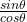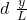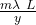## A diffraction grating is placed 1.00 m from a viewing screen. Light from a hydrogen lamp goes through the grating. A hydrogen spectral line

Question

A diffraction grating is placed 1.00 m from a viewing screen. Light from a hydrogen lamp goes through the grating. A hydrogen spectral line with a wavelength of 656 nm is seen 60.0 cm to one side of the center. Then, the hydrogen lamp is replaced with an unknown lamp. A spectral line is seen on the screen 36.4 cm away from the center. What is the wavelength of this spectral line

in progress 0
6 months 2021-08-27T20:38:43+00:00 1 Answers 3 views 0

## Answers ( )

λ = 396.7 nm

Explanation:

For this exercise we use the diffraction ratio of a grating

d sin θ = m λ

in general the networks works in the first order m = 1

we can use trigonometry, remembering that in diffraction experiments the angles are small

tan θ = y / L

tan θ == sin θ

sin θ = y / L

we substitute= m λ

with the initial data we look for the distance between the lines

d =d = 1 656 10⁻⁹ 1.00 / 0.600

d = 1.09 10⁻⁶ m

for the unknown lamp we look for the wavelength

λ = d y / L m

λ = 1.09 10⁻⁶ 0.364 / 1.00 1

λ = 3.9676 10⁻⁷ m

λ = 3.967 10⁻⁷ m

we reduce nm

λ = 396.7 nm